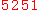### Scatter Plots and Simple Linear Regression

A scatter diagram is an extremely simple statistical tool used to show a relationship between two variables. It is often combined with a simple linear regression line used to fit a model between the two variables. To illustrate this, I collected some data on a recent trip to the La Brea Tar Pits.

The La Brea Tar Pits, located in Los Angeles, are a natural formation where tar seeps to the surface. These pits have existed for thousands of years, forming a natural trap contributing to the early demise of many an animal unlucky enough to stray into the tar. Since the 1940s, the pits have been excavated resulting in the discovery of many fossils, including Mammoths, Wolf, Bear, and some other lesser known animals such as the American Lion and American Camel.Most of the current activity and excavation revolve around Pit 91. On my trip to La Brea, the scientists and researchers were actively working in Pit 91. Below is a photo of Pit 91 along with the data I chose to use to introduce a scatter plot.In the picture, the number of specimens recovered by year is depicted. These results in table form are…

 Year # Specimens 2004 2,452 2005 2,826 2006 2,189 2007 3,388

A simple scatter plot places a dot where each year intersects the number of specimens collected in that year.Another useful feature of scatter plots is that they are easily completed by simple linear regression in the placement of a regression line through the data. In Microsoft Excel, this can be done by inserting a trendline. This results in the plot below.Many have questioned how this line is calculated and what it means. This line is a model that comes from the simple linear regression of the data. The basis of this line is the equation we all learned in high school, which is y=mx+b, where y = # of specimens and x is the year. In this specific case, the equation for the line is y=217.1x-432680 or # Specimens = 217.1*year-432680.

The calculation of the line is a little more complex. The values for m and b (slope and intercept, respectively) are calculated from the data using the simple linear regression.This equation guarantees that the distance from each data point to the line squared is minimized. Graphically, this can be seen in the plot below.The distance from each point to the line is the error, depicted in the graph above as e1, e2, e3, and e4. The linear regression line returns the values of m (slope) and b (intercept) that reduce the sum of the errors squared. Put another way, m and b are calculated to minimize (e12+e22+e32+e42). Another name for simple linear regression is “least squares regression”, a name which describes the result of the tool.

While it is possible to fit a line through just about any data, that doesn’t mean you should. If there is no relationship between the year and the # of specimens, then we really shouldn’t show a model indicating that there is. One of the complimentary statistics with regression is a p Value, sometimes called a p(2-Tail). The regression output from SPC XL, below, has the regression statistics including the p Value for the variable Year.We can be (1-p Value)*100% certain that a change in year results in a change in the # of specimens found. The p Value for year is .461, which means that we are (1-.461)*100% or 54% confident that a change in year results in a change in the number of specimens found. At 54% confidence, we would normally not conclude that changing the variable year results in a change in the # of specimens found.

A scatter chart with a regression model is an excellent tool which can be used to depict the relationship between two variables. When used properly, you can get not only a visual representation but a mathematical model that relates the two variables. However, be careful not to depict a relationship where one does not exist.

 昵   称： 内   容： 验证码：